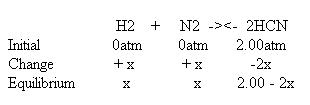# Pressure equilibrium/Gibbs free energy question

• MaxwellKraft
In summary, the first part of the conversation is about an equilibrium and the partial pressures at equilibrium. The second part is about calculating the Gibbs free energy for the reverse reaction. I was struggling with the entropy for the reactant in the second part, but I am now hopefully able to solve it.f

#### MaxwellKraft

Hey everyone. First let me say thanks for reading this. I am doing some practice exams to prepare for a Chemistry exam on Thursday, but I’m finding I don’t know the material very well. The problem I am having the most difficulty with right now has two parts, one relating to pressure equilibriums, and one relating to Gibbs free energy.

Part 1

Consider the following equilibrium.

H2(g) + N2(g) + 2C(s) -><- 2HCN(g) Kp = 3.4x10^-3

If the initial pressure of HCN is 2.00 atm, calculate the partial pressures of H2, N2, and HCN at equilibrium.
What I did:

The carbon is solid and does not affect equilibrium, and so the equilibrium expression is
Kp (3.4x10^-3 ) = HCN^2/H2 x N2

Then I set up an ice table.(I tried to make this in text but it I figured this would be easier)

This makes the equilibrium expression

3.4x10^-3 = 2.00 – 2x / x^2

This is where I got a little messed up. To solve this we have to use the quadratic equation, so I cross multiplied 3.4 x 10^3 x x^2, and 2.00 – 2x x (since 3.4x10^-3 is actually 3.4x10^-3/1), and got

3.4x10^-3x^2 = 2.00 – 2x

Which I rearranged to

3.4x10^-3x^2 + 2x -2.00 = 0

I then plugged this into the quadratic equation y = (+/- √2b – 4ac) / 2a

Where a = 3.4x10^-3, b = 2, and c = -2.00

My possible answers were 1.03 and -589.30. 1.03 was the only reasonable one, so I
concluded that at equilibrium the partial pressures of H2 and N2 were 1.03 atm, and the partial pressure of HCN was 0.97 atm. Does this seem correct? Also, is 1.03 the combined pressure of both N2 and H2? This would make their individual pressures ~0.52 atm, but since Kp is so low (< 1), shouldn't it favor reactants? I'm a little confused.

Part 2

For this part I have to calculate Gibbs free energy for the reverse reaction at 298K. The enthalpy for the forward reaction is +270kJ, so enthalpy of the reverse reaction is -270kj.The formula for Gibbs free energy (∆G) is

∆G= ∆H - T∆S

I’m given enthalpy (∆H) and temperature, but I need to calculate entropy. The formula for entropy is

∆S = ∑nS(products) - ∑mS(reactants)

Where n and m are stoichiometric coefficients, multiplied for the standard entropy for each species. However, this is where I get stuck. Since this is the reverse reaction, the reaction is

2HCN(g) -> H2(g) + N2(g) + 2C(s)

I have the entropies for H2(g) (130.684 J/K x mol), N2(g) (191.61 J/K x mol), and C(s) (5.740 J/K x mol), but not for HCN! I know I could look it up, but I’m not sure how to complete this calculating with the information that is given to me (I have the formula/constant sheet provided, and it doesn’t provide a standard entropy for HCN). I can calculate the entropies of the products (H2(g) + N2(g) + 2C(s), since the reaction was reversed), but I have no idea how to calculate the entropy of HCN (the reactant). I would appreciate any help with this problem.

Thanks in advance! Let me know if this isn’t clear enough and I will try to explain myself better.

I can help with part 1...

I then plugged this into the quadratic equation y = (+/- √2b – 4ac) / 2a

Did you use this formula:
(-b+/-$$\sqrt{b^{2}-4ac}$$)/(2a)
That equation you posted seems to be incorrect. Are you allowed to use a graphing calculator? If so, you can just plot 3.4x10^-3x^2 + 2x -2.00 and find out where it equals zero. That is the same as solving the quadratic.

Where a = 3.4x10^-3, b = 2, and c = -2.00

My possible answers were 1.03 and -589.30. 1.03 was the only reasonable one, so I
concluded that at equilibrium the partial pressures of H2 and N2 were 1.03 atm, and the partial pressure of HCN was 0.97 atm. Does this seem correct? Also, is 1.03 the combined pressure of both N2 and H2? This would make their individual pressures ~0.52 atm, but since Kp is so low (< 1), shouldn't it favor reactants? I'm a little confused.

The number that you solve for is equal to x in the ICE table. So, the answer is the pressure for H2 and N2 individually is 0.9983 atm.

My mistake. I did write the wrong equation, though I did use the correct one when I computed it.

So I guess that's right then. I'm still stuck on the 2nd part though.